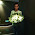## Copy List with Random Pointer

A linked list is given such that each node contains an additional random pointer which could point to any node in the list or null.
Return a deep copy of the list.

### Analysis:

This problem returns a new linked list, which has the same value and structure of the original one. First we can create a new linked list without considering the Random pointer, which is straight forward:  Scan every node in the original list and create the new list (line 21- 27 in the code below).

But how to keep the random pointer also correct ? If we only point the new random pointer to the original random pointer, which is not a deep copy (since the deletion of nodes in original list will delete the new one as well).  So, how to memorize the relative position of the random node to the current node? Firstly I think to use the length from head node to the random node. For each node, I stored the position of its random node, same position node in the new list is the random node for the node in the new list. This idea can work, but is not efficient. For every node you have to search from the start to the end to find the random, the total complexity is O(n^2). Can we quickly locate the position of the node?  Yes!  Hash map!  A map with the node as key and node as the value can finish the job! We can use the original list node as the key, and the same node in the new list as the value.  Now the map[node_old] = node_new, therefore the node_new->random = map[node_old->random]. In this way, the complexity decreases to O(n).

### Code:

/**
* Definition for singly-linked list with a random pointer.
* struct RandomListNode {
*     int label;
*     RandomListNode *next, *random;
*     RandomListNode(int x) : label(x), next(NULL), random(NULL) {}
* };
*/
class Solution {
public:
RandomListNode *copyRandomList(RandomListNode *head) {
// IMPORTANT: Please reset any member data you declared, as
// the same Solution instance will be reused for each test case.
map<RandomListNode*,RandomListNode*> mp; //map <originalNode, newNode>
mp.clear();
RandomListNode *res=new RandomListNode(0);
RandomListNode *q=res;

while (p){
RandomListNode *tmp = new RandomListNode(p->label);
q->next = tmp;
mp[p]=tmp;
p=p->next;
q=q->next;
}
q=res->next;
while (p){
if (p->random==NULL){
q->random==NULL;
}else{
q->random = mp[p->random];
}
p=p->next;
q=q->next;
}
return res->next;
}
};


1.line 32 : q->random==NULL ???

2.I have another solution without using hashmap.

public class Solution {
public RandomListNode copyRandomList(RandomListNode head) {
if(head == null) return null;

RandomListNode i = head, n, t;

while (i != null) {
n = i.next;
RandomListNode copy = new RandomListNode(i.label);

i.next = copy;
copy.next = n;
i = n;
}

//now assign random pointers
while(i!=null){
if(i.random!=null)
i.next.random = i.random.next;
i = i.next.next;
}

//now recover the original structure
i = head; n = i.next;
RandomListNode foo = n;
while(i!=null){
i.next = n.next;
i = i.next;
n.next = i==null?null:i.next;
n = n.next;
}
return foo;
}
}

U can also find recursion solution here: http://traceformula.blogspot.com/2015/07/copy-list-with-random-pointers.html

3.I have another solution without using hashmap.

public class Solution {
public RandomListNode copyRandomList(RandomListNode head) {
if(head == null) return null;

RandomListNode i = head, n, t;

while (i != null) {
n = i.next;
RandomListNode copy = new RandomListNode(i.label);

i.next = copy;
copy.next = n;
i = n;
}

//now assign random pointers
while(i!=null){
if(i.random!=null)
i.next.random = i.random.next;
i = i.next.next;
}

//now recover the original structure
i = head; n = i.next;
RandomListNode foo = n;
while(i!=null){
i.next = n.next;
i = i.next;
n.next = i==null?null:i.next;
n = n.next;
}
return foo;
}
}

U can also find recursion solution here: http://traceformula.blogspot.com/2015/07/copy-list-with-random-pointers.html